Published: 15 August 2014

# Identification of stiff inclusion in circular composite plate based on quaternion wavelet analysis of modal shapes

Andrzej Katunin1
1Institute of Fundamentals of Machinery Design, Silesian University of Technology, Gliwice, Poland
Views 44

#### Abstract

The inspection of composite structures is an important process during quality control and their operation in various conditions. Modern industrial requirements of structural condition assessment demand the evaluation of defects using non-destructive and non-contact methods, which allow for detection, precise localization and identification of defect. From a great variety of non-destructive methods the vibration-based ones seem to be effective, simple and low-cost. In order to improve the effectiveness of defects assessment the wavelet-based signal processing technique could be applied. In this paper the modal analysis of a composite circular plate with an inclusion was carried out in order to acquire the modal shapes of vibration. The acquired modal shapes were processed using quaternion wavelet transform. The application of this transform leads to the better directional selectivity than classical discrete wavelet transform. Obtained results allow for detection and identification of location and dimensions of inclusion. The sensitivity analysis of considering particular modal shapes was carried out and described. Due to the effectiveness of the proposed approach it can be applied in laboratory testing as well as industrial quality control and non-destructive testing.

## 1. Introduction

Recently, the polymer composites are used for structural design in many branches, primarily in aircraft, aerospace, automotive and ship building industries. Due to the strategy of replacement of important and responsible elements made of conventional materials by polymeric composites one achieves the outstanding stiffness and strength properties of these elements with simultaneous significantly decreased weight with consequent fuel saving and performance improvement. However, it is necessary to develop appropriate methods and techniques for structural assessment of these materials, which will be sensitive to particular types of defects and damages and allow for efficient and low-cost testing them.

A great variety of non-destructive testing (NDT) methods applicable for composites allows for efficient damage assessment; however, some of these methods requires expensive testing equipment and often limited to laboratory conditions. One of groups of the oldest and low-cost methods is the group of methods based on modal analysis. The acquisition of natural frequencies of vibration and modal shapes has insignificant diagnostic capabilities, however, the application of advanced signal processing methods to the modal data allows for considerable improvement of damage detection, localization and identification processes.

The efficient signal processing of modal shapes is fundamental, when the modal data is used for the structural assessment. The signal processing techniques should be sensitive to local changes of stiffness in order to detect various types of defects and damages. Such criterion could be successfully fulfilled during application of wavelet-based signal processing techniques due to a very high sensitivity of them to singularities and discontinuities of processed signals. This approach was being intensively developed by the numerous research groups over the last decade. Considering the requirements of practical NDT the wavelet-based techniques were extended to the two-dimensional (2D) problems. During the wavelet analysis one should consider several factors, which have significant influence of local changes detectability, accuracy of localization and identification. The main of them are the type of the wavelet transform (WT) and the type of wavelet applied to a problem.

The application of 2D WTs to the structural damage detection and identification problems was presented and discussed in several studies. Such studies were introduced by Chang and Chen , where 2D continuous wavelet transform (CWT) with Gabor wavelets was applied for damage detection and localization in rectangular plates. The analysis was performed on the numerical data. Then, the authors of  presented a study of crack detection and identification in a rectangular plate using 2D CWT with tensor product symlets of order 6. The analysis was also based on numerical data, the method sensitivity analysis to the crack position and depth was performed. Probably the first experimental study using WT was presented by Rucka and Wilde . The authors tested a square plate made of polymethyl metacrylate (PMMA) with an artificial spatial damage using 2D CWT with Gaussian and reversed biorthogonal wavelets. Results of further studies in this area by several research groups confirmed the effectiveness of 2D CWT-based approach [4-6], however, it was proven in previous author’s studies [7, 8] that the CWT-based approach is redundant and could be substituted by application of discrete wavelet transform (DWT) with B-spline wavelets. A review of various WT-based algorithms, selection of wavelets and other parameters of WT during structural damage identification in composites was presented in .

The most of studies in the area of wavelet-based structural damage assessment refer to the rectangular-shaped structures. During the wavelet analysis the procedures are performed in the Cartesian coordinates. Nevertheless, many of engineering structures are characterized by the circular-shaped geometry and the WT in Cartesian coordinates could not be directly applied in these cases. However, based on previous studies , one can apply the WT adapted to the polar coordinates or simply transform data in polar coordinates to Cartesian ones, perform the WT-based analysis and transform the resulted data back to polar coordinates.

An application of 2D DWT has some disadvantages, which have a significant influence on damage detection and identification. The main problems are the lack of shift invariance and angular selectivity of 2D DWT, i.e. the resulted sets of detail coefficients have preferred directions (horizontal and vertical) and a diagonal set of detail coefficients, which have not straightforward interpretation . In order to enhance the directional selectivity and thus the accuracy of damage detection and identification one can use the complex dual-tree approach. Such an approach is used in complex discrete wavelet transform (CDWT) proposed by Kingsbury . This transform uses complex wavelet basis functions, whose real and imaginary parts are the Hilbert transform pairs. This leads to the fact that the resulted coefficients are almost shift invariant in spite of DWT, where the small shift of a signal cause significant disturbances of wavelet coefficient energy. The natural extension of CDWT to 2D space is the quaternion wavelet transform (QWT) introduced by Chan et al. in , which is based on quaternion algebra and generalized dual-tree approach. An application of QWT with respect to 2D DWT guarantees the better selectivity and, in spite of pure dual-tree approach, QWT is not redundant with respect to 2D DWT (the 2D dual-tree analysis is 4× redundant with respect to 2D DWT). The QWT has found numerous applications primarily in image processing problems: multiresolution analysis [13, 14], segmentation  and classification [16, 17] and seems to be suitable for the damage assessment problems.

In this study a composite circular plate with internal sector-shaped inclusion was tested. The measurements were carried out using laser Doppler vibrometers in order to acquire the modal shapes of vibration of a plate. The modal shapes were then processed using wavelet-based algorithm using QWT. Obtained results show a great effectiveness of the proposed algorithm, which implies the possibility of its application in industrial structural assessment problems as well. The advantages of QWT with respect to 2D DWT were presented basing on the investigated problem as a comparative study. The sensitivity analysis allows for a selection of modal shapes, which contain the most of diagnostic information and omit those containing a noise without significant contribution of diagnostic information.

## 2. Theoretical preliminaries and identification procedure

### 2.1. Algebra of quaternions and quaternion wavelet transform

The algebra of quaternions $\mathbf{H}$ is a hypercomplex algebra extended from the algebra of complex numbers $\mathbf{C}$. The quaternion $q$ is a set of four real numbers (basis elements) ${x}_{0}$, ${x}_{1}$, ${x}_{2}$, ${x}_{3}$ with one real ${e}_{0}$ and three unreal units ${e}_{1}$, ${e}_{2}$, ${e}_{3}$, ${e}_{1}^{2}={e}_{2}^{2}={e}_{3}^{2}=-{e}_{0}=-1\text{.}$ The commutation is described as follows: $q={e}_{0}{x}_{0}+{e}_{1}{x}_{1}+{e}_{2}{x}_{2}+{e}_{3}{x}_{3}\text{.}$ Since $\mathbf{H}$ is not commutative the multiplication rules are different than in $\mathbf{C}$ and can be presented as follows:

1
${e}_{1}{e}_{2}=-{e}_{2}{e}_{1}={e}_{3},{e}_{2}{e}_{3}=-{e}_{3}{e}_{2}={e}_{1},{e}_{1}{e}_{3}=-{e}_{3}{e}_{1}={e}_{2}$

The conjugate of $q$ is defined as ${q}^{*}={x}_{0}-{e}_{1}{x}_{1}-{e}_{2}{x}_{2}-{e}_{3}{x}_{3}$ and the magnitude is denoted as $\left|q\right|=\sqrt{q{q}^{*}}$. Since the QWT is a hypercomplex transform one should define the 2D analytic signal ${f}_{Q}\left(x,y\right)$, which can be presented as follows:

2
${f}_{Q}\left(x,y\right)=f\left(x,y\right)+{e}_{1}{\mathcal{H}}_{x}\left(f\left(x,y\right)\right)+{e}_{2}{\mathcal{H}}_{x}\left(f\left(x,y\right)\right)+{e}_{3}{\mathcal{H}}_{x}{\mathcal{H}}_{y}\left(f\left(x,y\right)\right),$

where ${H}_{i}$ denotes 1D Hilbert Transform applied along $x$ and $y$ coordinates.

The decomposition of a 2D analytical signal using QWT has a form:

3
$f\left(x,y\right)={a}_{l}f\left(x,y\right)+\sum _{l=1}^{n}\left[{d}_{l,1}f\left(x,y\right)+{d}_{l,2}f\left(x,y\right)+{d}_{l,3}f\left(x,y\right)\right],$

where $a$ and $d$ denote the sets of approximation and detail components, respectively, $l$ is a current decomposition level and $n$ is a number of considered decomposition levels. Same as 2D DWT, QWT could be considered as sets of quaternion filters or combinations of tensor products of 1D complex subbands. The quaternion scaling function $\mathrm{\Phi }\left(x,y\right)$ and three wavelet functions ${\mathrm{\Psi }}_{i}\left(x,y\right)$ can be then presented in the form :

4
$\mathrm{\Phi }\left(x,y\right)={\varphi }_{h}\left(x\right){\varphi }_{h}\left(y\right)+{e}_{1}{\varphi }_{g}\left(x\right){\varphi }_{h}\left(y\right)+{e}_{2}{\varphi }_{h}\left(x\right){\varphi }_{g}\left(y\right)+{e}_{3}{\varphi }_{g}\left(x\right){\varphi }_{g}\left(y\right),$
${\mathrm{\Psi }}_{1}\left(x,y\right)={\psi }_{h}\left(x\right){\varphi }_{h}\left(y\right)+{e}_{1}{\psi }_{g}\left(x\right){\varphi }_{h}\left(y\right)+{e}_{2}{\psi }_{h}\left(x\right){\varphi }_{g}\left(y\right)+{e}_{3}{\psi }_{g}\left(x\right){\varphi }_{g}\left(y\right),$
${\mathrm{\Psi }}_{2}\left(x,y\right)={\varphi }_{h}\left(x\right){\psi }_{h}\left(y\right)+{e}_{1}{\varphi }_{g}\left(x\right){\psi }_{h}\left(y\right)+{e}_{2}{\varphi }_{h}\left(x\right){\psi }_{g}\left(y\right)+{e}_{3}{\varphi }_{g}\left(x\right){\psi }_{g}\left(y\right),$
${\mathrm{\Psi }}_{2}\left(x,y\right)={\psi }_{h}\left(x\right){\psi }_{h}\left(y\right)+{e}_{1}{\psi }_{g}\left(x\right){\psi }_{h}\left(y\right)+{e}_{2}{\psi }_{h}\left(x\right){\psi }_{g}\left(y\right)+{e}_{3}{\psi }_{g}\left(x\right){\psi }_{g}\left(y\right),$

where the subscripts $h$ and $g$ of 1D scaling and wavelet function denote the pulse responses of low-pass and high-pass quaternion filters, respectively.

### 2.2. Processing algorithm

The analysis is based on acquired modal shapes of vibration of a structure. Each of the extracted shapes was transformed using QWT, which resulted in four sets of coefficients. The meaningful diagnostic information is stored in the detail coefficients sets and thus only these sets were used for further processing. According to the fact that the magnitudes in acquired modal shapes are in dependence with the magnitudes of resulted detail coefficients it is necessary to consider more than one modal shape because the damage could be located in the low-magnitude region on a given modal shape and would not be detectable. From the other hand, a large number of considered modal shapes could influence negatively on detection and proper identification of a damage due to the presence of noise resulted from measurements and the transform. Considering the same dependence between magnitudes of vibration and detail coefficients it is evident that the magnitudes of detail coefficients obtained from high-order modal shapes will be lower than those obtained from low-order modal shapes. The analyses in the area show [18, 19] that the high-order modes usually contain more diagnostic information about the structure condition. Following this it is suitable to normalized considered modal shapes. After normalization procedure it is essential to emphasize the damaged regions for visualization purposes. The previously used approach presented in  is based on the determination of Euclidean norm of all obtained sets of detail coefficients. Such approach allows for effective damage detection, localization and identification, which will be presented further basing on experimental data.

## 3. Experimental

### 3.1. Specimen preparation

The tested specimen was manufactured in the Institute of Lightweight Structures and Polymer Technology, TU Dresden, as 12-layered laminated glass fibre-reinforced circular plate with lay-up given by the structural formula ${\left[\text{0}/\text{60}/\text{-60}\right]}_{2S}$. The material properties of the plate are similar as those described in . The diameter of a plate was 500 mm and a total thickness was 2.1 mm. For the mounting purpose a circular hole was cut in the centre of a plate with diameter of 60 mm. During the lamination process the sector-shaped PTFE tape was inserted between sixth and seventh layers. The inclusion has the radial dimensions same as the radial dimensions of a plate and the angular dimension of 20°. The scheme of a plate with inclusion was presented in Fig. 1.

Fig. 1The scheme of a tested plate and the experimental setup during the modal analysis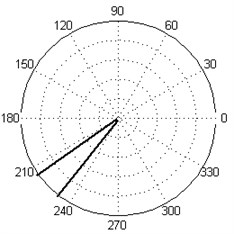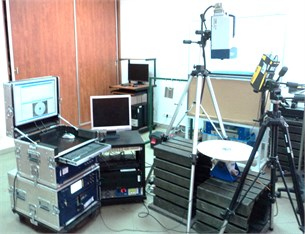### 3.2. Modal analysis

In order to acquire modal shapes the laser Doppler vibrometers (LDV) were used. The scanning was performed using Polytec® PSV-400 scanning LDV connected with a vibrometer controller OFV-5000 with built-in velocity and displacements decoder. The plate was clamped in the centre using a steel disc with a diameter of 78 mm. On the available area of a plate the net of scanning points was defined in the vibrometer-dedicated software PSV v.8.7 in polar coordinates with dimensions of 64×64 points in radial and angular directions, which resulted in 4096 measurement points. The excitation of a plate was performed by the electrodynamic shaker TIRA® TV-51120 connected with the power amplifier TIRA® BAA-500. A pseudo-random noise signal was used as excitation of a tested plate. For the acquisition of a reference signal the Polytec® PDV-100 point LDV was used, which was focused on the steel disc in the centre of a plate. In order to ensure better focusing of a laser beam the plate and a steel disc was covered by Helling® anti-glare spray dedicated for laser scanning. The experimental setup is shown in Fig. 1.

The frequency bandwidth was defined in the range of 0÷1250 Hz with the resolution of 0.78125 Hz. For each point defined on the measurement net the frequency response function (FRF) was obtained. Five averaging cycles were defined for each measurement point. From the resulted FRF for the whole net of measurement points 10 modes were selected for further analysis (see Fig. 2). The frequency values, corresponded with the selected modes, are presented in Table 1.

Fig. 2FRF obtained from the modal analysis of a plate with marks of the selected modes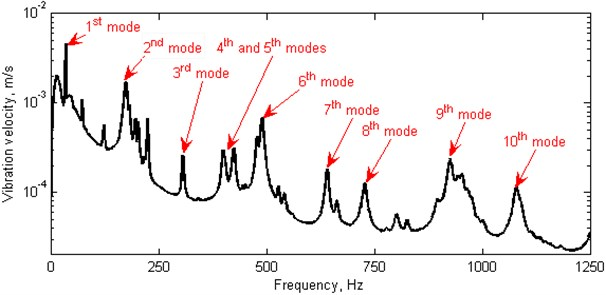Table 1Frequencies corresponded to the modal shapes

 Modal shape 1st 2nd 3rd 4th 5th Frequency, Hz 34.375 173.4375 306.25 400.78125 425 Modal shape 6th 7th 8th 9th 10th Frequency, Hz 489.84375 640.625 727.34375 925.78125 1078.90625

## 4. Results and discussion

### 4.1. Identification of inclusion

Obtained datasets were firstly transformed from polar coordinate system to Cartesian one in order to perform the wavelet analysis. The square 64×64 element matrices were used for the QWT described above. Single-level decomposition was carried out according to Eq. (3). For the obtained sets of detail coefficients the normalization procedure was applied to the range of [0, 1] and then the Euclidean norm was calculated for all of the sets following the formula:

5
$D=\sqrt{\sum _{M=1}^{10}{\left(\sum _{i=1}^{3}{d}_{i}^{M}\right)}^{2}},$

where $M$ is a number of considered modes and $i$ is the number of detail coefficient sets. Then, the resulted $D$-coefficients were transformed back to the polar coordinate system.

In order to show the influence of considered modal shapes three cases were selected: in the first case $M=$1÷10, in the second case $M=$6÷10 and in the third case $M=$ 6, 8, 10. The resulted $D$-coefficients are presented in Fig. 3. The true location of the inclusion is marked by the black lines.

It could be noticed that in all cases presented in Fig. 3 the inclusion was detected and localized properly. Depending on a number of modal shapes considered in the analysis the results of identification of specific dimensions of an inclusion were different. It is caused by the influence of low-order modal shapes, where the values of velocity of vibrations have lower number of extrema and do not contain information about the singularities. In the case of high-order modal shapes the defect lies in the non-zero curvature regions and can be detected by the presented approach (in this case $M=$6÷10), which confirms the statement that the high-order modal shapes are characterized by better sensitivity to the structural changes and imperfections [18, 19]. Once the analysis for all modal shapes was performed, it is possible to select the modal shapes sensitive to the damage (in this case $M=$ 6, 8, 10), which gives accurate identification of the defect dimensions.

Fig. 3Results of inclusion identification during considering of various number of modal shapes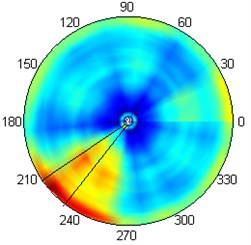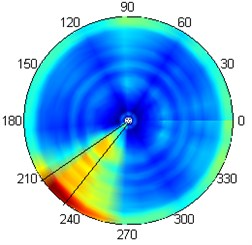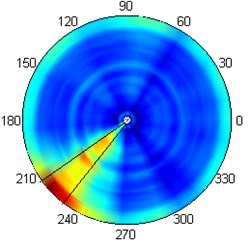### 4.2. Comparison of 2D DWT and QWT

In order to emphasize the advantages of QWT the acquired modal shapes were processed by 2D DWT with quadratic B-spline wavelets using the same post-processing approach given by Eq. (5) and compared with the results presented in Fig. 3. The results for mentioned cases ($M=$ 1÷10, $M=$ 6÷10 and $M=$ 6, 8, 10) are presented in Fig. 4.

Fig. 4Results of inclusion identification using 2D DWT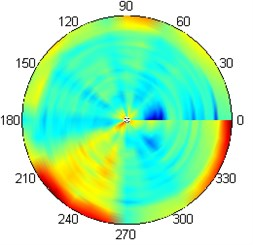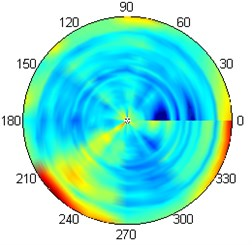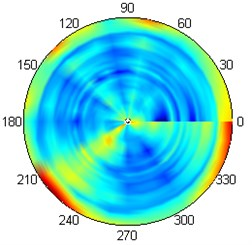The inclusion is still detectable in these cases, however due to the fact that 2D DWT is a single-tree WT the localization ability of an inclusion is worse than in the case of QWT (cf. Fig. 3 and Fig. 4). Due to the better directional selectivity of QWT the shape of inclusion is well recognized (see Fig. 3) in spite of the results using 2D DWT, where the angular set of coefficients is mainly detectible.

## 5. Conclusions

The quaternion wavelet transform was used for the identification of stiff inclusion in a circular composite plate based on analysis of modal shapes of vibrations of this plate. It was shown that the application of QWT guarantees the better directional selectivity and thus better sensitivity to the imperfections with respect to 2D DWT, which allows for improvement of a structural damage assessment algorithm. The analysis of sensitivity of particular modal shapes to imperfection confirmed the statement that the high-order modal shapes provide better identification than the low-order ones, which is in agree with the previous studies in this area. Due to the accurate results obtained during the analysis the proposed algorithm could be succesfully applied in industrial problems of damage assessment and quality control of composite structures.